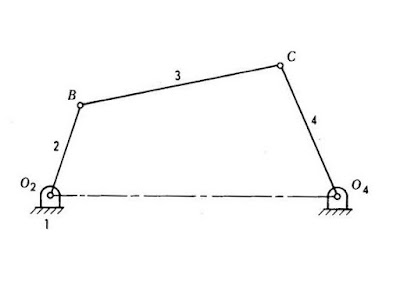In the theory of machine when we are familiar with basic mechanism, kinematic links, kinematic pairs, etc. There is very important topic that we should be aware of. Degree of freedom and kinematic constraint. These are very important criterion to consider during machine building. Degree of freedom and kinematic constraints are opposite things. But to understand one we have to understand another also. This Article mainly focused for kinematic constraints, so that would be elaborated here.four bar mechanism with constraints

What is the meaning of kinematic constraints is described in the following definition.

### Define constraint

kinematic constraints are the restrictions for a link in the motion of a machine.
Constraints are the inverse of degree of freedom. As the degree of freedom is about how many directions and axis an element can move.

What would be the inverse of it - How many direction and axis an element is restricted to move. This criteria will known as kinematic constraints.

Any element can move in three translatory direction and three rotational direction. So the maximum type of motions an element can have will be referred as degree of freedom and the maximum motion that an element is restricted for motion can be said as degree of constraints.

If a system has degree of constraints then it will hold the system rigidly. To make a body rigid the degree of constraints should be higher and the degree of freedom should be constrained.

Lower Pair and Higher Pair

What is kinematic Pair. What is its classification

### Types of constraints

There are mainly two categories of kinematic constants
1. Holonomic constraints
2. Non holonomic constraints

#### Holonomic constraints

These Constraints arise from joints cam joints, hinges, sliding elements etc. Holonomic constants also called geometric restrictions.These constraints are responsible for the construction of a system

#### Non holonomic constraints

The constraints which are applied on the movement velocity of any system  are called non holonomic constraints. The common examples of this type of constraints are, rolling without sliping, knife-egde constraint etc.

### Examples of kinematic constraints

Constraints are very basic criteria of mechanism formation. some basic examples are given below

#### Kinematic coupling

In the kinematic coupling all the translatory and rotational degree of freedom are constraints.

#### Kinematic chains

The primary body of a mechanism is called link. This link is rigid in nature. Links connected by kinematics pair are called kinematics chain. the degree of freedom of a kinematic chain can be calculated by formule. So the constraints in the kinematic chains are also measurable.

#### Kinematic pairs

Kinematics pairs are formed by creating a joint between links. There are many types of kinematic pairs. Kinematic pairs help the system to implement the constraints in a mechanism.

#### Rolling without slipping

When an object rolls in a surface without slipping, the velocity of the centre of mass will be cross product of angular velocity and a vector from the point of contact of the centre of mass.

### Constraints Equation

In the algebraic form the equation of kinematic constraints can be written as
F=F(q)
Where q Is the vector of body coordinates and f is the function of kinematic constraints.
On differentiation of this equation, vacation will become
•
F= Dv
Where D is the matrix and v denotes the velocity.

Again we differentiate this equation and it will be
••    •    •
F= Dv +Dv
•
here v Represent the acceleration.
let
•
J = -Dv

then                                             •
Dv = J
•
the equation can be solved in terms of v and v can be calculated by any generic method.

These equations are used to find out the constants of any system. Kinematic analysis are carried out with the help of these equations.

• Smooth motion in the machine system.
• Increasing the stability of mechanism.
• Increase rigidity.
• Better control on the motion of links of mechanism.

#### Conclusion

So in the analysis of kinematic system, kinematic constraints are very important criteria to consider. The analysis of kinematic constraints can provide better results to control the mechanism or motion in a system.

#### 1 comment:

1.Kya bat Soni bhai